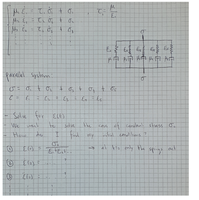# Finding initial conditions

#### Joa

##### New member
Hello,

I am trying to solve a differential equation corresponding to a model for visco-elastic material behavior. the model consists of 5 units of spring-dampers placed in parallel and should be solved for the strain $$\displaystyle \varepsilon(t)$$. The material is subject to a constant stress $$\displaystyle \sigma_0$$. I am able to come-up with a single fifth order differential equation but I am struggling to find the initial conditions. I have found the first initial condition as can be seen in the attached image, but don't know how to come-up with the remaining four conditions. Can anyone help me solve this problem?

Joa#### sergiokp

##### New member
I think that the key idea is that you can write a second order differential equation to describe the system. Anyway, I do not understand how you get those equations because whenever you use Newton's second law, since the acceleration appears, equations usually involve second derivatives.

#### Subhotosh Khan

##### Super Moderator
Staff member
I think that the key idea is that you can write a second order differential equation to describe the system. Anyway, I do not understand how you get those equations because whenever you use Newton's second law, since the acceleration appears, equations usually involve second derivatives.
For linear-elastic material ....................................stress = k*(strain)

For Visco-elastic Newtonian fluid.........................stress = k*(strain-rate)